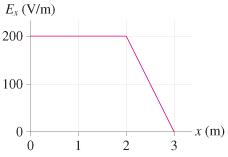# Problem: The figure is a graph of Ex. The potential at the origin is -300 V.What is the potential at x = 3.0 m?

###### FREE Expert Solution

The potential at 3.0 m is given by:

V3 - V0 = - area under the curve.

V3 - (-300) = - area under the curve.

84% (43 ratings)###### Problem Details

The figure is a graph of Ex. The potential at the origin is -300 V.What is the potential at x = 3.0 m?

Frequently Asked Questions

What scientific concept do you need to know in order to solve this problem?

Our tutors have indicated that to solve this problem you will need to apply the Electric Potential concept. You can view video lessons to learn Electric Potential. Or if you need more Electric Potential practice, you can also practice Electric Potential practice problems.

What professor is this problem relevant for?

Based on our data, we think this problem is relevant for Professor Markelz's class at UB.